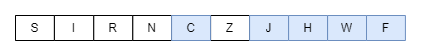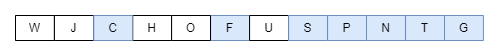# Lexicographically Smallest K-Length Subsequence From a Given String

## Introduction

Arrays and strings are the two data structures that form the basics of any programming language. A tight grip over these is essential to solving complex problems using advanced data structures. The questions of such topics are solved using other data structures, including stacksqueues, vectors, etc. To crack any interview, a solid grip over these is essential.

This blog will discuss a common problem to find the lexicographically smallest K-length subsequence from a given string

## Problem Statement

You have been provided with a string S, of length N, and an integer K. Your task is to determine the lexicographically smallest subsequence of length K from this string S. Also, it has been stated that K < N.

A sequence X is said to be a subsequence of sequence Y if X can be obtained from Y after several (maybe zero) elements are deleted. For example, XVTO is a subsequence of ZYXWVUTSRO

Example

S = SIRNCZJHWF

K = 5

The lexicographically smallest subsequence of length 5 is CJHWF.S = WJCHOFUSPNTG

K = 7

The lexicographically smallest subsequence of length 7 is CFSPNTG.## Brute Force Approach

### Algorithm

• We will generate all possible subsequences of the given string of length K and store them in a vector.
• Then we will sort the vector.
• The subsequence at the 0-th index will be our lexicographically smallest subsequence of length  K.

### Implementation in C++

``````// C++ program to find the lexicographically smallest K-length subsequence from a given string using brute force.
#include <iostream>
#include <vector>
#include <algorithm>
using namespace std;

// Vector to store all the possible subsequences of length K.
vector<string> allSubStr;

// Function to generate all possible subsequences of length K and store them in a vector.
void generate(string currStr, string inputStr, int ind, int k)
{
if (currStr.length() == k)
{
allSubStr.push_back(currStr);
return;
}
if (ind >= inputStr.length())
return;

// Recursively calling the function and including the current character into the current string.
generate(currStr + inputStr[ind], inputStr, ind + 1, k);

// Recursively calling the function without including the current character into the current string.
generate(currStr, inputStr, ind + 1, k);
}
int main()
{
string currStr, inputStr;
int k;

// Taking user input.
cout << "Enter the string: ";
cin >> inputStr;
cout << "Enter the value of k: ";
cin >> k;

// Calling the function to generate all subsequences of length 'K'.
generate(currStr, inputStr, 0, k);

sort(allSubStr.begin(), allSubStr.end());

cout << "The lexicographically smallest k-length subsequence is ";
cout << allSubStr;

return 0;
}
``````

### Implementation in Java

``````import java.io.*;
import java.util.*;
public class Main {
static ArrayList<String> allSubStr;
public static void main (String[] args) {
Scanner sc = new Scanner(System.in);
String currStr = "", inputStr;
int k;

// Taking user input.
System.out.println("Enter the string: ");
inputStr = sc.next();
System.out.println("Enter the value of k: ");
k = sc.nextInt();

allSubStr = new ArrayList<>();
// Calling the function to generate all subsequences of length 'K'.
generate(currStr, inputStr, 0, k);

Collections.sort(allSubStr);

System.out.println("The lexicographically smallest k-length subsequence is ");
System.out.println(allSubStr.get(0));
}
private static void generate(String currStr, String inputStr, int ind, int k) {
if (currStr.length() == k)
{
return;
}
if (ind >= inputStr.length())
return;

// Recursively calling the function and including the current character into the current string.
generate(currStr + inputStr.charAt(ind), inputStr, ind + 1, k);

// Recursively calling the function without including the current character into the current string.
generate(currStr, inputStr, ind + 1, k);
}
}``````

### Implementation in Python

``````
#List to store all the possible subsequences of length K
allSubStr=[]
def generate(currStr,inputStr,ind,k):

if(len(currStr)==k):
allSubStr.append(currStr)
return
if(ind>=len(inputStr)):
return
#Recursively calling the function and including the current character into the current string.
generate(currStr + inputStr[ind], inputStr, ind + 1, k)

#Recursively calling the function without including the current character into the current string
generate(currStr, inputStr, ind + 1, k)

if __name__ == '__main__':

#Taking user input.
inputStr=input("Enter the string:")
k=int(input("Enter the value of k:"))
currStr=""

#Calling the function to generate all subsequences of length 'K'.
generate(currStr, inputStr, 0, k)
allSubStr.sort()

print("The lexicographically smallest k-length subsequence is " + allSubStr)
``````

Input

``````Enter the string: sirnczjhwf
Enter the value of k: 5``````

Output

``The lexicographically smallest k-length subsequence is cjhwf``

### Complexity Analysis

Time Complexity

The time complexity is O(2N), where N is the length of the given string.

The recursive (see Recursion) function makes 2N calls to generate all possible subsequences of length K, so the time complexity is given by O(2N)

Space Complexity

The space complexity is O(N), where N is the length of the given string.

Since there are 2N  recursion calls and the size of our recursive tree is N, thus the space complexity is given by O(N).

## Stack Data Structure

### Algorithm

• In this approach, we will use a stack data structure to keep track of the characters in increasing order. At any string index, this stack will contain the smallest K-length subsequence up to that index.
• As you traverse the string, push the current character into the stack if it is empty.
• If not, iterate the string till the current element of the string, S[i]becomes smaller than the topmost element of the stack. Also, since the maximum size of the stack is K, you need to continuously pop off elements from the stack as well.
• If the stack size after the previous step is less than K, then push the current character into the stack.
• After this, the characters stored in the stack are printed in reverse order to obtain the required K-length subsequence.

### Implementation in C++

``````// C++ program to find the lexicographically smallest K-length subsequence from a given string.
#include <iostream>
#include <stack>
#include <algorithm>
using namespace std;

// Function to find the lexicographically smallest K-length subsequence from a given string.
void lexicographicallySmallestSubsequence(string &s, int k)
{
// Storing the length of the string.
int n = s.length();

// Initialising a variable to store the lexicographically smallest substring.
stack<char> ans;

// Traversing the string.
for (int i = 0; i < n; i++)
{
// In case the stack is empty.
if (ans.empty())
{
ans.push(s[i]);
}
else
{
// Iterating till the current character is less than the character at the top of the stack and checking if at least K characters remain in the rest of the string.
while ((!ans.empty()) && (s[i] < ans.top()) && (ans.size() - 1 + n - i >= k))
{
ans.pop();
}

// If the size of the stack is less than k, the character is pushed into it.
if (ans.empty() || ans.size() < k)
{
ans.push(s[i]);
}
}
}

// Declaring a variable to store the final result.
string result;

// Iterating till the stack becomes empty.
while (!ans.empty())
{
result.push_back(ans.top());
ans.pop();
}

// Reversing the string and printing it.
reverse(result.begin(), result.end());
cout << result;
}

int main()
{
string s;
cout << "Enter the string: ";
cin >> s;
int k;
cout << "Enter the value of k: ";
cin >> k;

// Calling the function and printing the answer.
cout << "The lexicographically smallest k-length subsequence is ";
lexicographicallySmallestSubsequence(s, k);

return 0;
}
``````

### Implementation in Java

``````
import java.io.*;
import java.util.*;
public class Main {
static ArrayList<String> allSubStr;
public static void main (String[] args) {
Scanner sc = new Scanner(System.in);
String s;
System.out.println("Enter the string: ");
s = sc.next();
int k;
System.out.println("Enter the value of k: ");
k = sc.nextInt();

// Calling the function and printing the answer.
System.out.println("The lexicographically smallest k-length subsequence is ");
lexicographicallySmallestSubsequence(s, k);
}
private static void lexicographicallySmallestSubsequence(String s, int k) {
// Storing the length of the string.
int n = s.length();

// Initialising a variable to store the lexicographically smallest substring.
Stack<Character> ans = new Stack<>();

// Traversing the string.
for (int i = 0; i < n; i++)
{
// In case the stack is empty.
if (ans.isEmpty())
{
}
else
{
// Iterating till the current character is less than the character at the top of the stack and checking if at least K characters remain in the rest of the string.
while ((!ans.isEmpty()) && (s.charAt(i) < ans.peek()) && (ans.size() - 1 + n - i >= k))
{
ans.pop();
}

// If the size of the stack is less than k, the character is pushed into it.
if (ans.empty() || ans.size() < k)
{
ans.push(s.charAt(i));
}
}
}

// Declaring a variable to store the final result.
String result = "";

// Iterating till the stack becomes empty.
while (!ans.isEmpty())
{
result = result + ans.pop();
}

// Reversing the string and printing it.
result = new StringBuilder(result).reverse().toString();

System.out.println(result);
}
}``````

### Implementation in Python

``````#Python program to find the lexicographically smallest K-length subsequence from a given string
#Function to find the lexicographically smallest K-length subsequence from a given string.
def lexicographicallySmallestSubsequence(s, k):

#Storing the length of the string.
n = len(s)
#Initialising a variable to store the lexicographically smallest substring.
ans=[]

#Traversing the string.
for i in range(n):
#In case the stack is empty.
if (len(ans) == 0):
ans.append(s[i])
else:
#Iterating till the current character is less than the character at the top of the stack and checking if at least K characters remain in the rest of the string
while (len(ans) > 0 and (s[i] < ans[len(ans) - 1]) and (len(ans) - 1 + n - i >= k)):
ans=ans[:-1]
#If the size of the stack is less than k, the character is pushed into it
if (len(ans) == 0 or len(ans) < k):
ans.append(s[i])

#Declaring a variable to store the final result
result=[]

#Iterating till the stack becomes empty
while(len(ans)>0):
result.append(ans[len(ans)-1])
ans=ans[:-1]

#Reversing the string and printing it
result=result[::-1]
result=''.join(result)
print("The lexicographically smallest k-length subsequence is " + result)

if __name__ == '__main__':
s=input("Enter the string:")
k=int(input("Enter the value of k:"))

#Calling the function and printing the answer
lexicographicallySmallestSubsequence(s,k)   ``````

Input

``````Enter the string: sirnczjhwf
Enter the value of k: 5``````

Output

``The lexicographically smallest k-length subsequence is cjhwf``

### Complexity Analysis

Time Complexity

O(N), where N is the length of the string.

The time complexity to push an element into the stack is given by O(1), and we are pushing N elements into the stack; the time complexity is given by O(N)

Space Complexity

The space complexity is O(K)where K is the length of the required output string.

The maximum number of characters that our answer stack can accommodate is K, so the space complexity is O(K).

### What is the time complexity of the push and pop operation?

The push and pop operations take place in constant i.e..O(1) time

### Why is the recursive approach not the most efficient one?

As the size of the string increases the cost for the computations in the recursive method increases exponentially as its time complexity is of the order 2N, therefore it would give the Time Limit Exceeded i.e., TLE error for inputs of larger lengths (≥20)

## Conclusion

So, this blog discussed the problem to find the lexicographically smallest K-length subsequence from a given string. To discover more, go to CodeStudio right now to practice problems and ace your interviews like a Ninja!

#### Recommended Problems

In today's competitive environment, memorizing a handful of questions isn't enough. So, take our mock tests to evaluate where you stand among your peers and what areas you need to work on. You can also check out some Basic String Problems as well as a few of the Guided Paths on CodeStudio

If you have any suggestions or recommendations, please leave them in the comments box.

Happy Coding!!